Everyday maths 1

Start this free course now. Just create an account and sign in. Enrol and complete the course for a free statement of participation or digital badge if available.

Free course

# 1 Around the edge

When might you need to work out how far it is around a flat shape?

You will need to know how far it is around the edge of a shape when you want to put a border around something, such as a wallpaper border around a room, or a brick wall around a patio. You might have thought of different examples.

The distance around any shape is called the perimeter. You can work out the perimeter by adding up all of the sides. The sides are measured in units of length or distance, such as centimetres, metres or kilometres.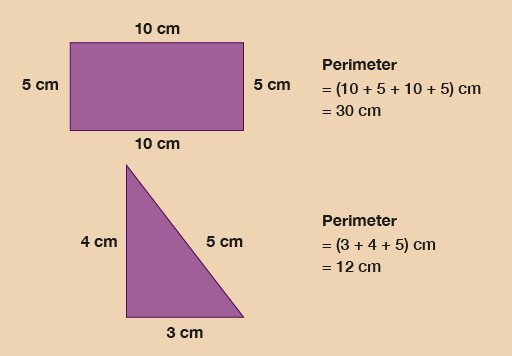Figure 1 Looking at perimeters

## Example: A length of ribbon

Have a look at the figure below to work out how much decorative ribbon you need to go around each shape.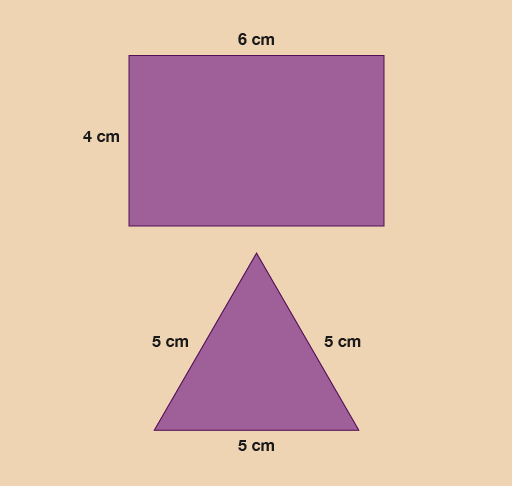Figure 2 Calculating the length of ribbon

### Method

You need to measure all the sides and add them together.

Hint: Opposite sides of a rectangle are the same length.

The sides of the rectangular box are:

6 + 6 + 4 + 4 = 20 cm

You will need 20 cm of ribbon.

The sides of the triangular box are:

5 + 5 + 5 = 15 cm

You will need 15 cm of ribbon.

## Example: A quicker calculation

So far when you have been working with perimeter you have added up all four sides, however there is a quicker way of calculating the perimeter. You may have recognised that all rectangles have two equal short sides and two equal long sides. Therefore you can then work out the perimeter by using each number twice.

(2 × long side) + (2 × short side) = perimeter

The long side is the length. The short side is the width.

(A square is a type of rectangle where all four sides are the same length. So to find out the perimeter of a square, you need to multiply the length of one side by 4.)

How many metres of lawn edging do you need to go around this lawn?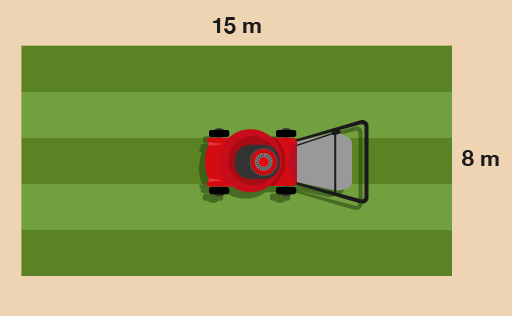Figure 3 A lawn

### Method

You need to work out twice the width, plus twice the length:

(2 × 15) + (2 × 8)

Once you’ve worked these out, it makes the answer to the question easier to get:

(2 × 15) + (2 × 8) = 30 + 16 = 46 m

Now try the following activity. Remember to check your answers once you have completed the questions.

## Activity 2: Finding the perimeter

1. You need to hang bunting around the tennis courts for the local championships. How much bunting do you need?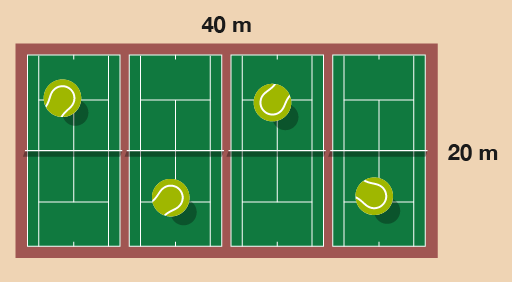Figure 4 Four tennis courts
1. How much tape does the police officer need to close off this crime scene?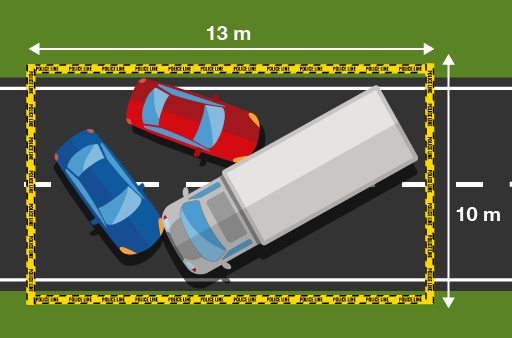Figure 5 A crime scene

1. The sides of the tennis courts are 20 m and 40 m.

• (2 × 20) + (2 × 40) = 40 + 80 = 120

So 120 m of bunting will be needed.

2. The sides of the crime scene are 13 m and 10 m.

• (2 × 10) + (2 × 13) = 20 + 26 = 46

So 46 m of police tape will be needed.

FSM_1